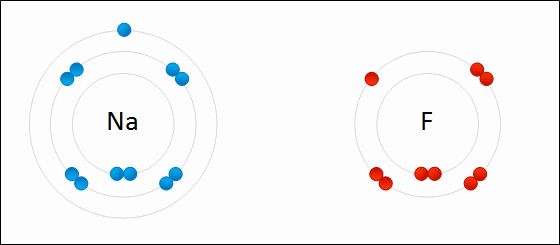## Formulas of Ionic Compounds

#### Learning Objective

• Generate the empirical formula of an ionic compound given its molecular constituents.

#### Key Points

• The overall ionic formula for a compound must be electrically neutral, meaning it has no charge.
• When writing the formula for the ionic compound, the cation comes first, followed by the anion, both with numeric subscripts to indicate the number of atoms of each.
• Polyatomic ions are a set of covalently bonded atoms that have an overall charge, making them an ion.
• Polyatomic ions form ionic bonds in the typical way, balancing so that the overall compound is electrically neutral.

#### Terms

• monatomic ionAn ion made of only one atom, for example Cl-.
• polyatomic ionA set of covalently bonded atoms that have an overall charge, making them an ion.

Ionic bonds are formed by the transfer of one or more valence electrons between atoms, typically between metals and nonmetals. The transfer of electrons allows the atoms to effectively achieve the much more stable electron configuration of having eight electrons in the outermost valence shell (octet rule). When sodium donates a valence electron to fluorine to become sodium fluoride, that is an example of ionic bond formation.Formation of sodium flourideThe transfer of electrons between two atoms to create two ions that attract each other because they are oppositely charged.

## Writing Ionic Formulas

Ionic compounds can be described using chemical formulas, which represent the ratios of interacting elements that are found in the ionic solid or salt. Ionic solids are typically represented by their empirical formulas. In formula notation, the elements are represented by their chemical symbols followed by numeric subscripts that indicate the relative ratios of the constituent atoms. The complete formula for an ionic compound can be determined by satisfying two conditions:

• First, the charge on the constituent ions can be determined based on the transfer of valence electrons necessary in order to satisfy the octet rule.
• Second, the cations and anions are combined in a way that produces a electrically neutral compound.

For example, in the reaction of calcium and chlorine, the compound is called calcium chloride. It is composed of Ca2+ cations and Cl anions; those ions are stable since they have filled valence shells. Its ionic formula is written as CaCl2, the neutral combination of these ions. Two chloride ions were needed in the final compound because calcium had a 2+ charge. To create a neutral compound, CaCl2, two 1- chloride ions were needed to balance out the 2+ charge from calcium.

## Polyatomic Ions

Polyatomic ions are a set of covalently bonded atoms that have an overall charge, making them an ion. For example, the hydroxide ion has the formula OH-1. Hydroxide is a compound made of oxygen and hydrogen that have been bound together. In the process of becoming a compound, hydroxide gained an extra electron from somewhere, making it OH-1. When creating ionic compounds with these polyatomic ions, treat them the same way as typical monatomic ions (only one atom).

For example, calcium hydroxide has the formula Ca(OH)2 because hydroxide has -1 charge and calcium has a 2+ charge. Two hydroxides were needed to balance off the +2 charge of calcium. The parentheses were used to indicate that OH was a polyatomic ion and came as a “package deal.” Two hydroxides couldn’t have been written O2H2 because that is a very different compound than (OH)2. Parentheses are always used when the compound contains multiples of the polyatomic ion.

Here is a list of common polyatomic ions:

• Ammonium, NH4+
• Carbonate, CO32-
• Bicarbonate, HCO3
• Cyanide, CN
• Phosphate, PO43-
• Hydroxide, OH
• Nitrate, NO3
• Permanganate, MnO4
• Sulfate, SO42-
• Thiocyanate, SCN
• Peroxide, O22-